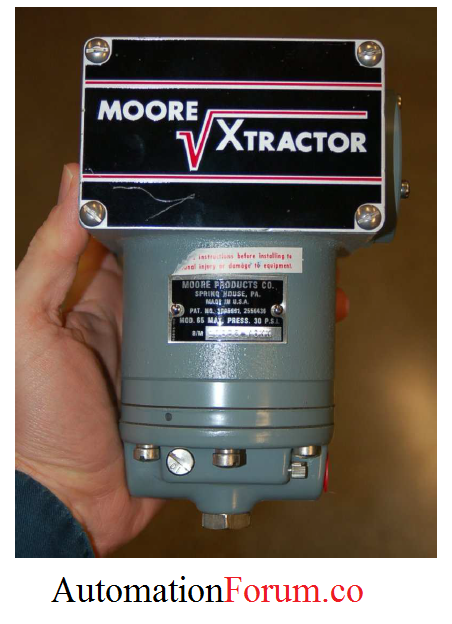# Square root extractor for DP flow measurement## Why use square root extractor for DP transmitter?

The DP flowmeters measure the differential pressure across the constrictions made in the flow channel. The differential pressure measured has nonlinear relation with flow through the pipeline. Differential pressure developed by a venturi, orifice plate, pitot tube, or any other acceleration-based flow element is proportional to the square of the flow rate. The square root extractor is used to convert the measured differential pressure into flow rate.When plotted on a graph, the relationship between flow rate (Q) and differential pressure (ΔP) is quadratic, like one-half of a parabola.

## Square root extractor:

The square root extractor is actually a kind of signal conditioner. So that we can have indicators, recorders and controllers that actually register linearly with the flow velocity, we must mathematically “condition” or “characterize” the pressure signal detected by the differential pressure transmitter to corresponding flow rate.

The modern solution to this problem is to incorporate the characterization of the square root signal inside the transmitter or inside the receiver instrument (eg indicator, recorder or controller). At the time of pneumatic instrumentation, this square root function was performed separately device called square root extractor. The traditional means of implementing the necessary signal characterization was to install a “square root” function relay between the transmitter and the flow indicator as shown below picture:The square root extractor is an electronic (or pneumatic) device that takes the square root of the signal from the flow transmitter and outputs a corresponding linear flow signal. Several methods are used in the construction of square root extractors.

The following table shows the ideal response of a pneumatic square root relay:The square root relationship is most evident in comparing the input and output percentage values. For example, at an input signal pressure of 6 PSI (25%), the output signal percentage will be the square root of 25%, which is 50% (0.5 = √0.25) or 9 PSI as a pneumatic signal.